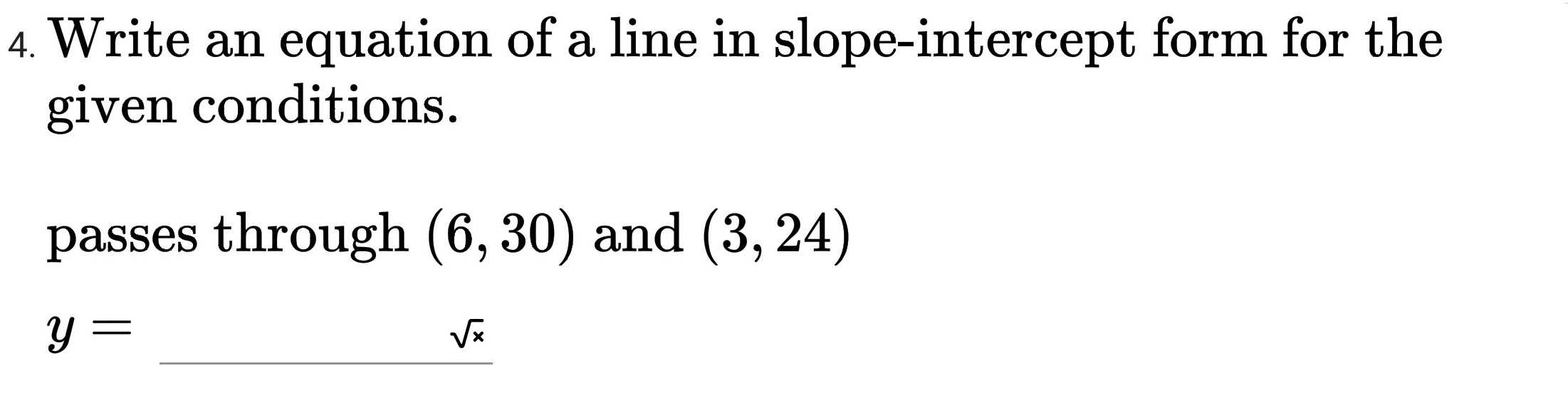### ¿Todavía tienes preguntas de matemáticas?

Pregunte a nuestros tutores expertos
Algebra
PreguntaWrite an equation of a line in slope-intercept form for the given conditions. passes through $$( 6,30 )$$ and $$( 3,24 )$$

$$y =$$

$$y= 2x+ 18$$